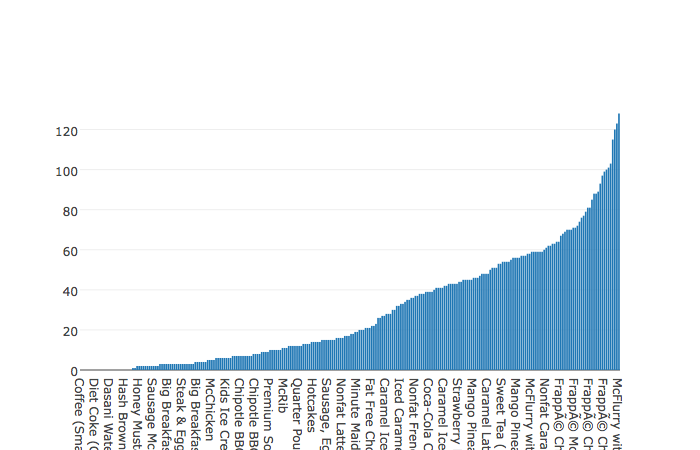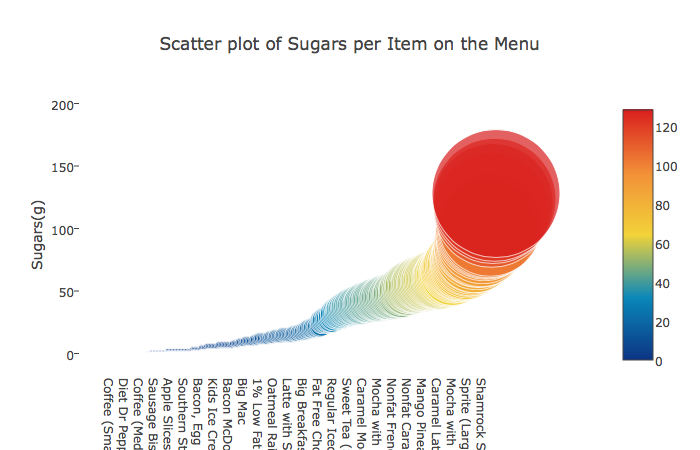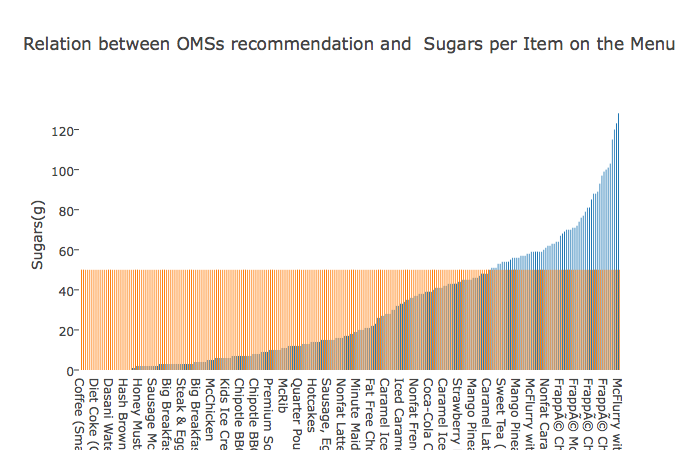# Sugars in McDonalds Menu’s Items

3 minute read

Recently I’ve found a data set in Kaggle which is composed with the nutrition facts of every item in McDonald’s Menu (Dataset).

I know for a while that the consumption of sugar in our life is way upon the one recommended by the health care organizations, so I wanted to analyzed how much sugar where in the McDonald menu’s items and which of them don’t have any kind of added sugar.

For this I’ve used a Jupyter Notebook with the following libraries: plotly and pandas. Here I’m going to explain the different steps I’ve followed, but the complete notebook could be checked in my Github Repository.

The information is in a cvs. First let’s load the information to see how it is structured:

``````menu = pd.read_csv('./menu.csv')
menu.head(10)
``````

With this we could see the information of the dataset. This means, the columns and the rows.

Ok, the one I’m interested in is sugar, so I’m going to create a new pandas data frame composed by the column with the item’s name and the amount of sugar, and Aldo I’m going to order them in an increasing order:

``````df_sugars = pd.DataFrame(columns=('Item','Sugars'))
df_sugars['Item'] = menu['Item']
df_sugars['Sugars'] = menu['Sugars']
print("Let's sort them by the amount of sugar they have in a ascending order: ")
df_sugars = df_sugars.sort_values('Sugars', ascending=[True])
print(df_sugars.head(10))
``````

So now that I have this, I want to check which are the menu items that don’t have any amount of sugar:

``````print("Number of items in the menu: "+str(len(menu.index)))
print("Number of items without sugar in the menu: "+str(len(df_sugars.loc[df_sugars['Sugars'] == 0])))
print(df_sugars.loc[df_sugars['Sugars'] == 0])
``````

And I obtain the following result:

``````Number of items in the menu: 260
Number of items without sugar in the menu: 25
Item  Sugars
145                Coffee (Small)       0
99              Kids French Fries       0
96             Small French Fries       0
81   Chicken McNuggets (20 piece)       0
114             Diet Coke (Small)       0
115            Diet Coke (Medium)       0
116             Diet Coke (Large)       0
117             Diet Coke (Child)       0
122        Diet Dr Pepper (Small)       0
123       Diet Dr Pepper (Medium)       0
124        Diet Dr Pepper (Large)       0
98             Large French Fries       0
80   Chicken McNuggets (10 piece)       0
79    Chicken McNuggets (6 piece)       0
136           Dasani Water Bottle       0
137              Iced Tea (Small)       0
138             Iced Tea (Medium)       0
139              Iced Tea (Large)       0
140              Iced Tea (Child)       0
78    Chicken McNuggets (4 piece)       0
146               Coffee (Medium)       0
38                     Hash Brown       0
147                Coffee (Large)       0
125        Diet Dr Pepper (Child)       0
97            Medium French Fries       0
``````

So only 25 elements of 260, which means that only the 9.61% of the items in McDonalds doesn’t have any amount of sugar. Now, let’s do the plot to see this graphically, for this I’m going to use the Plotly library:

``````print("Let's start with the bar chart")

data = [go.Bar(
y = df_sugars['Sugars'].values,
x = df_sugars['Item'].values,
)]

py.iplot(data, filename='basic-bar')
``````Also, I’m going to plot a scatter plot:

``````# Now let's plot a scatter plot
# This plot is based on the one made by Anisotropic:
# https://www.kaggle.com/arthurtok/super-sized-we-mcdonald-s-nutritional-metrics

trace = go.Scatter(
y = df_sugars['Sugars'].values,
x = df_sugars['Item'].values,
mode='markers',
marker=dict(
size= df_sugars['Sugars'].values,
#color = np.random.randn(500), #set color equal to a variable
color = df_sugars['Sugars'].values,
colorscale='Portland',
showscale=True
),
text = menu['Item'].values
)
data = [trace]

layout= go.Layout(
autosize= True,
title= 'Scatter plot of Sugars per Item on the Menu',
hovermode= 'closest',
xaxis=dict(
showgrid=False,
zeroline=False,
showline=False
),
yaxis=dict(
title= 'Sugars(g)',
ticklen= 5,
gridwidth= 2,
showgrid=False,
zeroline=False,
showline=False
),
showlegend= False
)
fig = go.Figure(data=data, layout=layout)
py.iplot(fig,filename='scatterChol')
``````The OMS tell that the max amount of sugar per day should be 50g. Let’s see the items of the menu go over this threshold:

``````# First let's add a new column to the dataframe, all equal to 50
df_sugars['Amount of Sugar recommended (g)'] = 50

# Let's plot them

trace1 = go.Bar(
y = df_sugars['Sugars'].values,
x = df_sugars['Item'].values,
name='Sugars(g)'
)
trace2 = go.Bar(
y = df_sugars['Amount of Sugar recommended (g)'].values,
x = df_sugars['Item'].values,
name='Recommended value of sugar OMS (g)'
)

data = [trace1, trace2]
layout = go.Layout(
barmode='group'
)

layout= go.Layout(
autosize= True,
title= 'Relation between OMSs recommendation and  Sugars per Item on the Menu',
hovermode= 'closest',
xaxis=dict(
showgrid=False,
zeroline=False,
showline=False
),
yaxis=dict(
title= 'Sugars(g)',
ticklen= 5,
gridwidth= 2,
showgrid=False,
zeroline=False,
showline=False
),
showlegend= False
)
fig = go.Figure(data=data, layout=layout)
graph = py.iplot(fig, filename='grouped-bar')
``````So as you could see, there’s a lot of items in the menu that are bad for our health for not saying all of them. For seeing the items in a more detailed way, you could check the notebook because the plots are interactive.

I hope you like this short analysis I’ve made. Check the repository in Github! :)

Tags:

Updated: